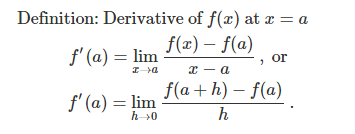# Calculus – Let’s begin to understand the world…Calculus is a branch of mathematics that deals with the study of change, motion, and rates of change. It is a powerful tool that has played a significant role in shaping the modern world and continues to be essential in many fields of study. From physics and engineering to economics and finance, calculus is used to understand and describe the world around us.
The origins of calculus can be traced back to the 17th century, when mathematicians such as Isaac Newton and Gottfried Wilhelm Leibniz independently developed the concepts of calculus. Their work laid the foundation for the field, and since then, calculus has evolved and grown to become one of the most important branches of mathematics.
One of the most significant contributions of calculus is its ability to describe change and motion in a precise and quantitative manner. Calculus allows us to understand how things change over time, and how different variables are related to one another. This makes it an essential tool for understanding the natural world and for solving real-world problems.
One of the most notable applications of calculus is in the field of physics. Calculus is used to understand the laws of motion and the behavior of matter and energy. It allows us to calculate the force and acceleration of objects, and to understand how they move and behave in different conditions. This is crucial in the design and development of modern technology, such as airplanes and power plants.
Another important application of calculus is in engineering. Engineers use calculus to optimize the design of complex systems, such as bridges and buildings. It is also used in the design of cars, smartphones and many other products. This makes calculus an essential tool for the development of modern technology.
Calculus is also used in the field of economics, particularly in the study of supply and demand, production costs and consumer behavior. It is also used to predict future trends in population growth and to understand the dynamics of financial markets.
To understand calculus, it is necessary to have a strong grasp of algebra and precalculus topics, such as functions and trigonometry. These concepts are fundamental to understanding limits, derivatives, and integrals, which are the building blocks of calculus.
Calculus is divided into two main branches: differential calculus and integral calculus. Differential calculus deals with the study of rates of change and slopes of curves, while integral calculus deals with the accumulation of quantities and the determination of areas under and between curves. Together, these two branches make up the field of calculus.
At the end, it’s worth mentioning that calculus has a subfield called “ordinary differential equations” (ODE) which is used to model the behavior of systems over time. ODE’s are widely used in physics, engineering, and other fields to model the behavior of systems over time. They are used to model the spread of disease, the movement of a missile, and many other real-world problems.
In conclusion, calculus is a powerful tool that has played a significant role in shaping the modern world. From physics and engineering to economics and finance, calculus is used to understand and describe the world around us. It’s important to have a strong grasp of algebra and precalculus topics, such as functions and trigonometry, to fully understand calculus. Understanding calculus is an essential tool for the development of modern technology and solving real-world problems.